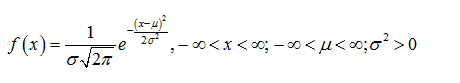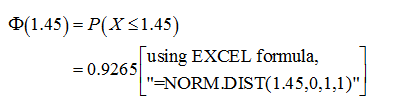# Let X be a standard normal random variable so that X∼N(0,1). For this problem you may want to refer to the table provided on Canvas. Recall, that Φ(x) denotes the standard normal CDF.(a) Find Φ(1.45).(b) Find x, such that Φ(x) = 0.4.(c) Based on the fact that Φ(1.645) = 0.95 find an interval in which X will fall with 95% probability.(d) Find another interval (different from the one in (c)) into which X will fall with 95% probability. Hint you may want to consider the symmetric properties of the standard normaldistribution.(e) Find an interval with lower bound -1.9 into which X will fall with 95% probability.(f) Find an interval with upper bound 2.1 into which X will fall with 95% probability.(g) Among all possible intervals into which X falls with 95% probability, find the shortest one.

Question
1 views
Let X be a standard normal random variable so that X∼N(0,1). For this problem you may want to refer to the table provided on Canvas. Recall, that Φ(x) denotes the standard normal CDF.
(a) Find Φ(1.45).
(b) Find x, such that Φ(x) = 0.4.
(c) Based on the fact that Φ(1.645) = 0.95 find an interval in which X will fall with 95% probability.
(d) Find another interval (different from the one in (c)) into which X will fall with 95% probability. Hint you may want to consider the symmetric properties of the standard normal
distribution.
(e) Find an interval with lower bound -1.9 into which X will fall with 95% probability.
(f) Find an interval with upper bound 2.1 into which X will fall with 95% probability.
(g) Among all possible intervals into which X falls with 95% probability, find the shortest one.
check_circle

Step 1

Note:

Hey there! Thank you for posting the question. Since there are multiple subparts are posted, according to our policy, we have solved first 3 sub parts for you. If you need help with any specific subpart, then please submit that subpart only or specify the subpart in your message.”

Since the normal table is not given, we have calculated the probability value using Excel.

Step 2

The normal distribution:

A continuous random variable X is said to follow normal distribution with mean µ and standard deviation σ if the probability density function of X is,Step 3

a)

Here, X is a standard normal variable.

The required probability is calculated as follows:...

### Want to see the full answer?

See Solution

#### Want to see this answer and more?

Solutions are written by subject experts who are available 24/7. Questions are typically answered within 1 hour.*

See Solution
*Response times may vary by subject and question.
Tagged in

### Statistics